# Types Of Angles

In geometry, there are various types of angles, based on measurement. The names of basic angles are Acute angle, Obtuse angle, Right angle, Straight angle, reflex angle and full rotation. An angle is geometrical shape formed by joining two rays at their end-points. An angle is usually measured in degrees.

There are various types of angles in geometry. Angles form the core part of the geometry in mathematics. They are the fundamentals that eventually lead to the formation of the more complex geometrical figures and shapes.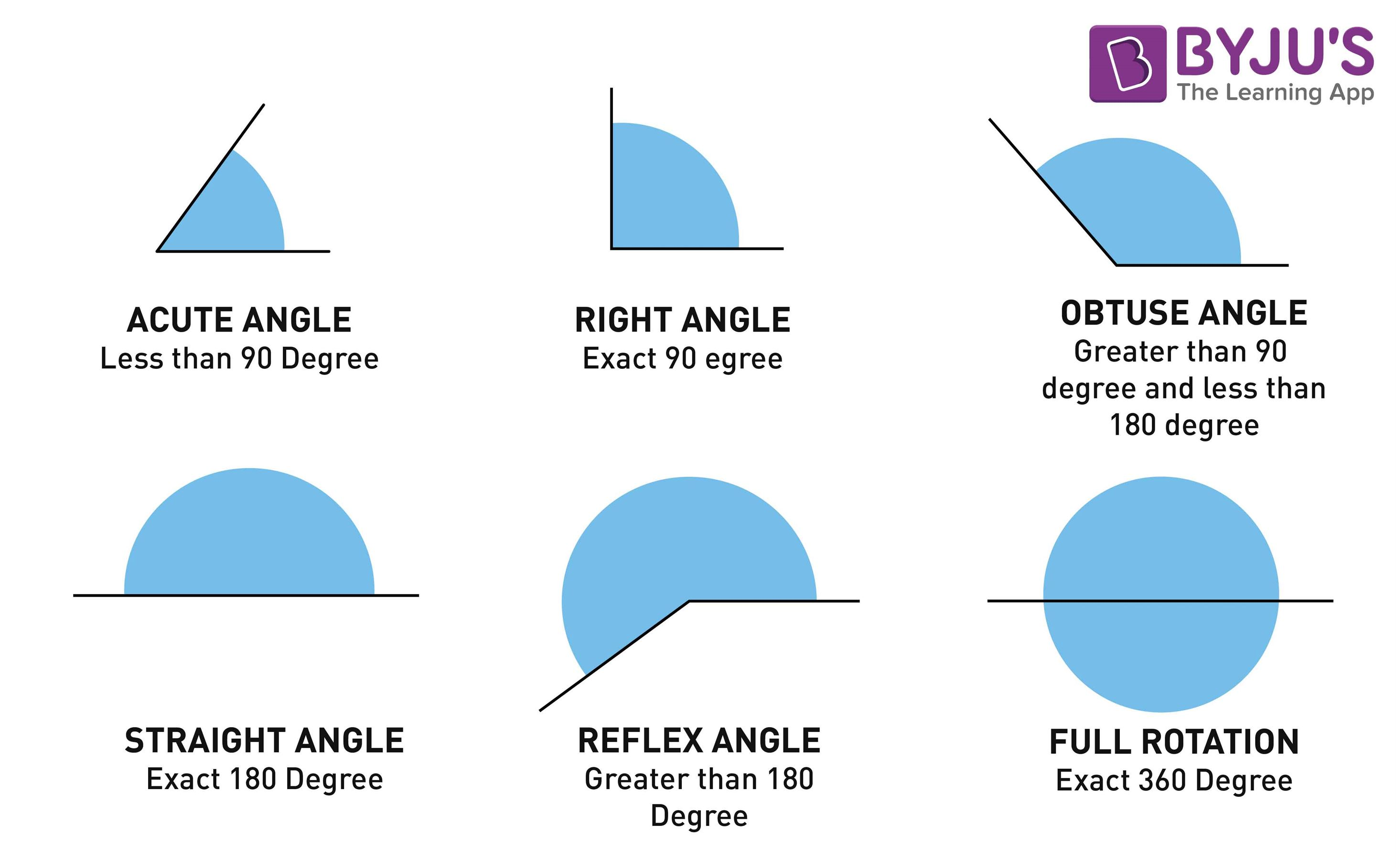Types Of Angles in Geometry

## What are Angles

When two rays combine with a common endpoint and the angle is formed. The two components of an angle are “sides” and “vertex”. The side can be categorized into terminal sides and initial sides (or vertical sides) as shown in the image below.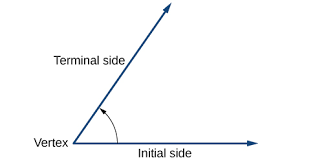Representation of an Angle

These two rays can combine in multiple fashions to form the different types of angles in mathematics. Let us begin by studying these different types of angles in geometry.

### Parts of Angle

• Vertex – Point where the arms meet.
• Arms – Two straight line segments form a vertex.
• Angle – If a ray is rotated about its endpoint, the measure of its rotation is called angle between its initial and final position.

#### For More Information On Parts Of An Angle, Watch The Below Video:## Classification of Angles

Angles can be classified into two main types:

• Based on Magnitude
• Based on Rotation

## Six Types of Angles

In Maths, there are mainly 5 types of angles based on their direction. These five angle types are the most common ones used in geometry. These are:

• Acute Angles
• Obtuse Angles
• Right Angles
• Straight Angles
• Reflex Angles
• Full Rotation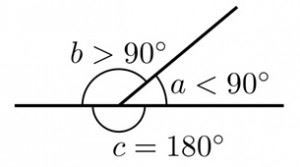Angle Types Based on Magnitude

The images above illustrate certain types of angles.

### Acute Angle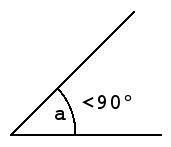Acute Angle

An acute angle lies between 0 degree and 90 degrees, or in other words; an acute angle is one that is less than 90 degrees. The figure above illustrates an acute angle.

### Obtuse Angle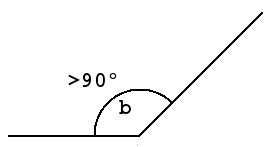Obtuse Angle

An obtuse angle is the opposite of an acute angle. It is the angle which lies between 90 degrees and 180 degrees or in other words; an obtuse angle is greater than 90 degrees and less than 180 degrees. The figure above illustrates an obtuse angle.

### Right Angle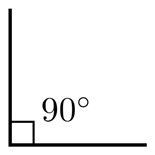Right Angle

A right angle is always equal to 90 degrees. Any angle less than 90 degrees is an acute angle whereas any angle greater than 90 degrees is an obtuse angle. The figure above illustrates a right angle or a 90-degree angle.

### Straight Angle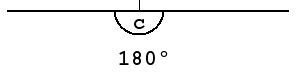Straight Angle

A straight angle is 180 degrees when measured. The figure above illustrates a straight angle or a 180-degree angle. You can see that it is just a straight line because the angle between its arms is 180 degrees.

### Reflex Angle

Since this measurement is less than 90 degrees, the arms form an acute angle. But what about the angle on the other side? What is the larger angle that is complementary to the acute angle called? It is called a reflex angle. The image below illustrates a reflex angle.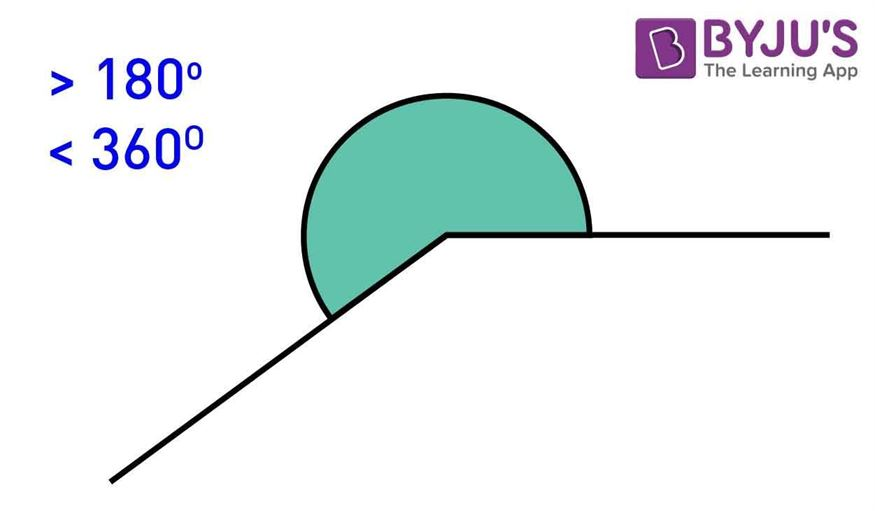Reflex Angle

Any angle that has a measure which is greater than 180 degrees but less than 360 degrees (which coincides with 0 degrees) is a reflex angle.

### Full Rotation

An angle equal to 360 degrees is called full rotation or full angle. It is formed when one of the arms takes a complete rotation to form an angle.

## Angle Types Based on Rotation

Based on the direction of measurement or the direction of rotation, angles can be of two types:

• Positive Angles
• Negative Angles

### Positive Angles

Positive angles are those angles which are measured in a counterclockwise direction from the base. In most cases, positive angles are used to represent angles in geometry. From the origin, if an angle is drawn in the (+x, +y) plane, it forms a positive angle.

### Negative Angles

Negative angles are those angles which are measured in a clockwise direction from the base. From the origin, if an angle is drawn towards the (x, -y) plane, it forms a negative angle.

## Pair of Angles

When two angles are paired, then there exist different angles, such as

• Complementary angles
• Supplementary angles
• Linear Pair
• Vertically Opposite angles
• ### Complementary and Supplementary Angles

Apart from the aforementioned types, there are two more angle types which are complementary angles and supplementary angles. If the sum of two angles is equal to 180°, then they are supplementary angles, and if the sum is equal to 90°, then they are called complementary angles.

### Linear Pair

When the non-common arms of adjacent angles are just opposite to each other, or they extend in the opposite direction, then they are called linear pairs. By linear it is clear that they form a straight line.

When two angles are connected with one common arm and have one common vertex and also the non-common arms are either side of the common arm, then they are called adjacent angles.

### Vertical Angles

When two lines intersect each other at a single point (called vertex), then the angle formed on either side of the common vertex is called vertical angles or vertically opposite angles.

## Angles Formed By Transversal

A line that cuts or intersects two or more lines at different points, is called a transversal. It is therefore, there are angles formed at the point of intersection. They are:

• Interior angles
• Exterior angles
• Pairs of Alternate interior angles
• Pairs of Alternate exterior angles
• Pairs of Corresponding angles
• Pairs of interior angles on the same side of the transversal
• ### Video Lesson on Types of Angles## Frequently Asked Questions – FAQs

Q1

### What are the six different angles in geometry based on measurement?

The six different angles in geometry based on magnitude are:
Acute angle, Obtuse angle, Right angle, Straight angle, Reflex angle and full angle
Q2

### What is an acute angle in geometry? Give examples.

The angle that measures less than 90 degrees is called acute angle.
Examples: 30°, 45°, 60°, 85° are acute angles.
Q3

### What is obtuse angle? Give an example.

The angle that measures greater than 90 degrees is obtuse. For example, 145° is an obtuse angle.
Q4

### What is reflex angle?Give an example.

Reflex angle is an angle that measures more than 180° and is less than 360°. For example, 270° is a reflex angle.
Q5

### What do you mean by zero angle?

When both the arms of an angle overlap each other and measure the angle of 0°, then it is called the zero angle.
Q6

### Is obtuse angle and reflex angle same?

Obtuse angle is different from reflex angle because obtuse lies between 90 degrees and 180 degrees but reflex is alway more than 180 degrees.
Test your Knowledge on Types Of Angles